Short Notes: Atoms and Molecules

# Short Notes: Atoms and Molecules - Science Class 9

 Table of contentsLaws of Chemical CombinationDalton's Atomic TheoryMoleculeWriting Chemical FormulaeMole ConceptDefinition of MoleMolar Mass## Laws of Chemical Combination

Given by Lavoisier and Joseph L. Proust as follows:

### Law of Conservation of Mass

Mass can neither be created nor destroyed in a chemical reaction.
Example:
A + B → C + D
Reactants → Products
Mass of reactants = Mass of products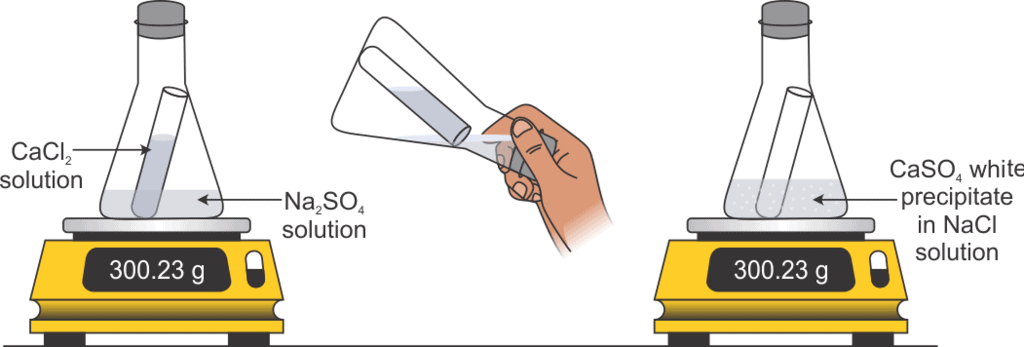Mass of Reactants = Mass of Products

### Law of Constant Proportions

In a chemical substance, the elements are always present in definite proportions by mass.

Example: In water, the ratio of the mass of hydrogen to the mass of oxygen is always 1:8 respectively.

These laws lacked explanation. Hence, John Dalton gave his theory about the nature of matter. He said that the smallest particles of matter are called ‘atom’.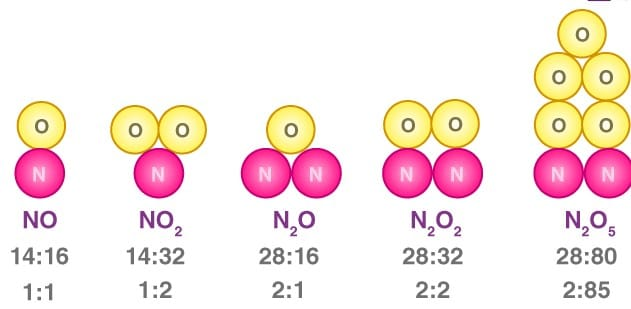An illustration describing the mass ratio of elements in a few compounds.

## Dalton's Atomic Theory

###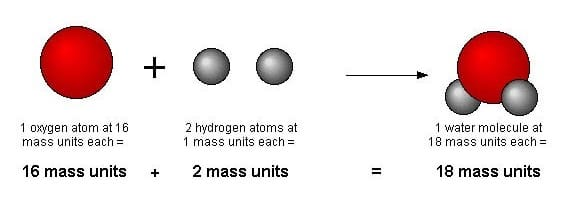• Every matter is made up of very small or tiny particles called atoms.
• Atoms are not divisible and cannot be created or destroyed in a chemical reaction.
• All atoms of a given element are the same in size, mass and chemical properties.
• Atoms of different elements are different in size, mass and chemical properties.
• Atoms combine in the ratio of small whole numbers to form compounds.
• The relative number and kinds of atoms are constant in a given compound.
• Atom Atoms are the smallest particles of an element that can take part in a chemical reaction.

Question for Short Notes: Atoms and Molecules
Try yourself:Which of the following is not a postulate of Dalton's atomic theory?

### Size of an Atom

Atomic radius is measured in nanometres.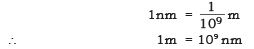Atomic radii of hydrogen atom = 1 × 10–10 m.

### Symbols of Atoms

(a) Symbols for some elements as proposed by Dalton: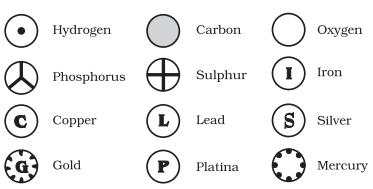Symbols for Elements as Proposed by Dalton(b) Symbols of some common elements: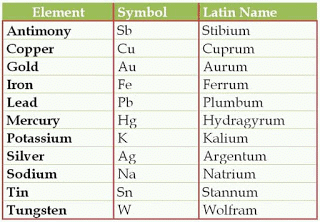## Molecule

• It is the smallest particle of an element or a compound that can exist independently.
• Molecules of an element constitute the same type of atoms.
• Molecules may be monoatomic, di-atomic or polyatomic.
• In molecules of compounds atoms of different elements join together in definite proportions and constitutes a different type of atoms.

### Atomicity

The number of atoms constituting a molecule is known as its atomicity.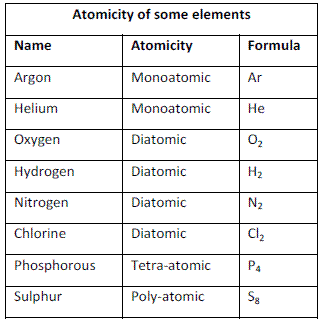### Ions

• The charged particles (atoms) are called ions, they are formed by attaining a positive charge or negative charge on them.
• A negatively charged ion is called anion (Cl).
• A positively charged ion is called a cation (Na+).

### Valency

• The combining capacity of an element is known as its valency. Valency is used to find out how an atom of an element will combine with the atom of another element to form a chemical compound. (Every atom wants to become stable, to do so it may lose, gain or share electrons.)
(i) If an atom consists of 1, 2 or 3 electrons in its valence shell then its valency is 1, 2 or 3 respectively,
(ii) If an atom consists of 5, 6 or 7 electrons in the outermost shell, then it will gain 3, 2 or 1 electron respectively and its valency will be 3, 2 or 1 respectively.
(iii) If an atom has 4 electrons in the outermost shell then it will share these electrons and hence its valency will be 4.
(iv) If an atom has 8 electrons in the outermost shell then its valency is 0.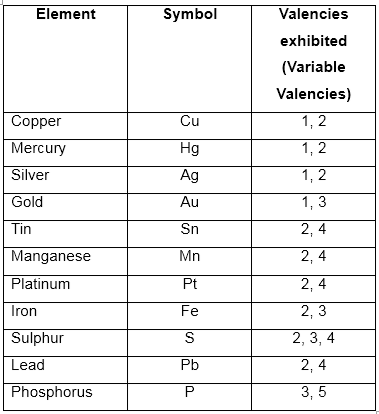• Some elements show more than one valency hence, termed variable valency.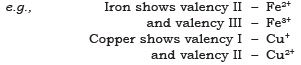Question for Short Notes: Atoms and Molecules
Try yourself:The chemical symbol for sodium is

## Writing Chemical Formulae

The chemical formula of a compound is a symbolic representation of its composition.

### Rules

• The valencies or charges on the ion must balance.
• Metal and non-metal compound should show the name or symbol of the metal first.
Example: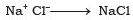• If a compound consists of polyatomic ions. The number of ions present in the compound is indicated by enclosing the formula of ion in a bracket and writing the number of ions outside the bracket.
Example: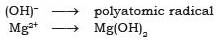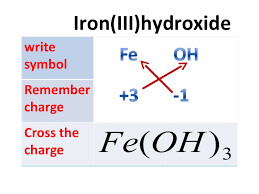### Chemical Formula of Some Simple Compounds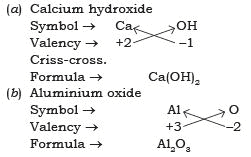### Molecular Mass

It is the sum of the atomic masses of all the atoms in a molecule of the substance. It is expressed in the atomic mass unit (u).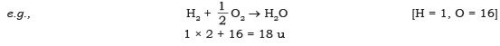### Formula Unit Mass

It is the sum of the atomic masses of all atoms in a formula unit of a compound. The constituent particles are ions.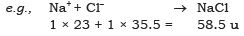## Mole Concept

### Definition of Mole

It is defined as one mole of any species (atoms, molecules, ions or particles) is that quantity in number having a mass equal to its atomic or molecular mass in grams. 1 mole = 6.022 × 1023 in number

## Molar Mass

mass of 1 mole → is always expressed in grams and is also known as gram atomic mass.
1u of hydrogen has → 1 atom of hydrogen
1g of hydrogen has → 1 mole of hydrogen
= 6.022 × 1023 atoms of hydrogen

The document Short Notes: Atoms and Molecules | Science Class 9 is a part of the Class 9 Course Science Class 9.
All you need of Class 9 at this link: Class 9

## Science Class 9

66 videos|352 docs|97 tests

## FAQs on Short Notes: Atoms and Molecules - Science Class 9

 1. What are the laws of chemical combination?Ans. The laws of chemical combination are a set of principles that describe how elements combine to form compounds. The three main laws are: 1. Law of Conservation of Mass: It states that in a chemical reaction, the total mass of the reactants is equal to the total mass of the products. This law implies that matter cannot be created or destroyed in a chemical reaction, only rearranged. 2. Law of Definite Proportions: It states that a compound always contains the same elements in the same fixed proportions by mass. Regardless of the amount of the compound, the ratio of the masses of the elements remains constant. 3. Law of Multiple Proportions: It states that when two elements form more than one compound, the masses of one element that combine with a fixed mass of the other element are in ratios of small whole numbers.
 2. What is Dalton's Atomic Theory?Ans. Dalton's Atomic Theory, proposed by John Dalton in the early 19th century, laid the foundation for modern atomic theory. The main postulates of Dalton's Atomic Theory are: 1. All matter is composed of tiny, indivisible particles called atoms. 2. Atoms of the same element are identical in mass, size, and chemical properties. Atoms of different elements have different properties. 3. Atoms combine in whole number ratios to form compounds. 4. Chemical reactions involve the rearrangement of atoms, but no atoms are created, destroyed, or transformed into atoms of another element. 5. Compounds are formed when atoms of different elements combine in fixed ratios. Dalton's Atomic Theory provided a framework for understanding the behavior of matter at the atomic level.
 3. What is atomic mass?Ans. Atomic mass refers to the mass of an individual atom of an element. It is determined by the total number of protons and neutrons in the atom's nucleus. Atomic mass is usually expressed in atomic mass units (amu) or unified atomic mass units (u). The atomic mass of an element is often represented as a weighted average of the masses of its naturally occurring isotopes, taking into account their relative abundance. The atomic mass is an important characteristic of an element as it helps in calculating the molar mass and stoichiometry in chemical reactions.
 4. What is a molecule?Ans. A molecule is the smallest particle of a substance that retains all the chemical properties of that substance. It is composed of two or more atoms held together by chemical bonds. Molecules can be made up of atoms of the same element (e.g., oxygen molecule - O2) or different elements (e.g., water molecule - H2O). The atoms in a molecule are arranged in a specific geometric structure, which determines the molecule's properties and behavior. Molecules are the basic building blocks of compounds and play a crucial role in chemical reactions.
 5. What is the mole concept?Ans. The mole concept is a fundamental concept in chemistry that relates the amount of a substance to its mass. It is based on the idea that the number of particles (atoms, molecules, or ions) in a substance can be counted in terms of moles. One mole is defined as the amount of substance that contains the same number of particles as there are atoms in exactly 12 grams of carbon-12. This number is known as Avogadro's number (6.022 × 10^23). The mole concept allows chemists to perform calculations involving the mass, volume, and number of particles of a substance, which is essential for understanding stoichiometry, empirical formulas, and reaction calculations.

## Science Class 9

66 videos|352 docs|97 testsExplore Courses for Class 9 examSignup to see your scores go up within 7 days! Learn & Practice with 1000+ FREE Notes, Videos & Tests.
10M+ students study on EduRev
Track your progress, build streaks, highlight & save important lessons and more!
Related Searches

,

,

,

,

,

,

,

,

,

,

,

,

,

,

,

,

,

,

,

,

,

;